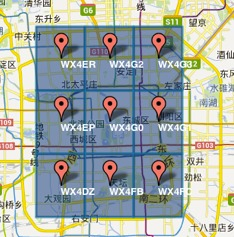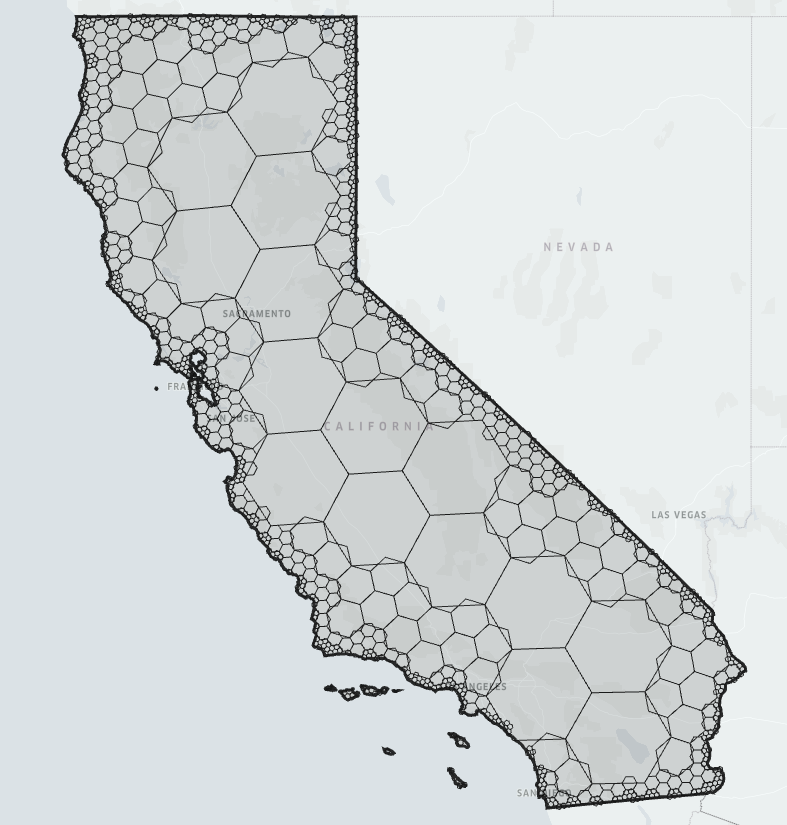## 举例## 空间划分

### 什么是空间划分

1. R-tree 为代表的动态单元
2. Geohash 为代表的静态单元

R-tree 简单来说是将空间划分为若干个不规则的边界框矩形的b+树索引，适用于面、线数据，查询时间复杂度为 O(n)。Geohash 简单来说是将二维的经纬度转换成字符串的四叉树线性索引，适用于点数据，查询时间复杂度为 O(log(N))### 静态单元对比### 什么是 H3H3 的前身其实是 DDGS(Discrete global grid systems) 中的 ISEA3H，其原理是把无限的不规则但体积相等的六棱柱从二十面体中心延伸，这样任何半径的球体都会穿过棱镜形成相等的面积cell，基于该标准使得每一个地理单元的面积大小就可以保证几乎相同。

# 加载相关包
library(dggridR)
library(dplyr)

# 构建公里网格
dggs <- dgconstruct(spacing = 1000, metric = FALSE, resround = "down")

# 加载测试数据集
data(dgquakes)

# 获取每个震源中心对应的网格
dgquakes$cell <- dgGEO_to_SEQNUM(dggs, dgquakes$lon, dgquakes$lat)$seqnum

# 将 SEQNUM 转为网格中心
cellcenters <- dgSEQNUM_to_GEO(dggs, dgquakes$cell) # 获取每个单元的地震次数 quakecounts <- dgquakes %>% group_by(cell) %>% summarise(count = n()) # 获取地震网格单元边界 grid <- dgcellstogrid(dggs, quakecounts$cell, frame = TRUE, wrapcells = TRUE)

# 更新网格单元的地震次数
grid <- merge(grid, quakecounts, by.x = "cell", by.y = "cell")

# Normarlize 指标便于展示
grid$count <- log(grid$count)
cutoff <- quantile(grid$count, 0.9) grid <- grid %>% mutate(count = ifelse(count > cutoff, cutoff, count)) # 获取每个国家的多边形 countries <- map_data("world") # 绘制地图 p <- ggplot() + geom_polygon(data = countries, aes(x = long, y = lat, group = group), fill = NA, color = "black") + geom_polygon(data = grid, aes(x = long, y = lat, group = group, fill = count), alpha = 0.4) + geom_path(data = grid, aes(x = long, y = lat, group = group), alpha = 0.4, color = "white") + geom_point(aes(x = cellcenters$lon_deg, y = cellcenters$lat_deg)) + scale_fill_gradient(low = "blue", high = "red") p转化坐标系后： # 重新在球坐标上绘制 p + coord_map("ortho", orientation = c(-38.49831, -179.9223, 0)) + theme( axis.ticks.x = element_blank(), axis.ticks.y = element_blank(), axis.text.x = element_blank(), axis.text.y = element_blank() ) + labs(x = "", y = "", title = "Your data could look like this")可以看到此时在若干个六边形中存在五边形的情形。 在 H3 开源后，也可以使用 h3r 实现六边形的编码与解码： # 以亮马桥地铁站为例 devtools::install_github("scottmmjackson/h3r") #或者 devtools::install_github("harryprince/h3r", ref="bug-fix/Makefile") library(h3r) df <- h3r::getBoundingHexFromCoords(39.949958, 116.46343, 11) %>% # 单边长为24米 purrr::transpose() %>% purrr::simplify_all() %>% data.frame() df %>% bind_rows( df %>% head(1) ) %>% leaflet::leaflet() %>% leafletCN::amap() %>% leaflet::addPolylines(lng = ~lon, lat = ~lat)H3 中还提供了类似 S2 的六边形压缩技术，使得数据的存储空间可以极大压缩，在处理大规模稀疏数据时将体现出优势：## 渲染引擎 ### 什么是 Leaflet 在使用 Deck.gl 之前，业界主流的解决方案通常是另一个开源的轻量级地理数据可视化框架 Leaflet。Leaflet 经过十余年的积累已经拥有足够成熟的生态，支持各式各样的插件扩展。 序号 leaflet 插件 功能 1 leaflet 基础功能，几何元素CRUD，图层等，可结合 shiny，crosstalk 2 leaflet.opacity 透明度调节 3 leaflet.extras 高级功能, 包括热力图, 搜索, 米尺等 4 leaflet.esri 高级功能，ESRI插件 可结合 Arcgis 5 mapview 高级功能, 多图联动等 6 mapedit 高级功能，地图编辑 7 leafletCN 提供高德底图 虽然 Leaflet 功能强大，不过工业界的发展也暴露出一些新的问题。如何更好地支持诸如 轨迹、风向、三维空间、六边形网格的交互式可视化此前没有好的解决方案。好在近年来 Mapbox 和 Deck.gl 正在着手改变这一现状。 ### 什么是 Deck.gl Deck.gl 基于 WebGL 的大规模数据可视化框架，通过响应式编程和GPU并行加速的方式进行高效地 WebGL 渲染，与 Mapbox GL 深度结合能够呈现非凡的 3D 视觉效果。 下面是一个具体的例子，如何以Hexagon可视化百万个样本点： # 初始化 library(mapdeck) # 生成 百万数据样本点 df = data.frame(lat = rnorm(1000000,40,1),lng =rnorm(1000000,160,1)) # 以二维正态生成随机数据 # 渲染 mapdeck::mapdeck(style = "mapbox://styles/mapbox/dark-v9",token = "pk.eyJ1IjoidWJlcmRhdGEiLCJhIjoiY2poczJzeGt2MGl1bTNkcm1lcXVqMXRpMyJ9.9o2DrYg8C8UWmprj-tcVpQ") %>% mapdeck::add_hexagon(lon = "lng",lat="lat",data = df,elevation_scale = 1000)除了六边形之外 Deck.gl 也支持其他常见几何图形，比如 Grid、Arc、Contour、Polygon 等等。 更多信息可以见官方文档： https://crazycapivara.github.io/deckgl/### 地理仪表盘：结合 Shiny Deck.gl 结合 Shiny 后，可将可视化结果输出到仪表盘上,举个例子： library(mapdeck) library(shiny) library(shinydashboard) library(jsonlite) ui <- dashboardPage( dashboardHeader(), dashboardSidebar(), dashboardBody( mapdeckOutput( outputId = "myMap" ), sliderInput( inputId = "longitudes", label = "Longitudes", min = -180, max = 180, value = c(-90, 90) ), verbatimTextOutput( outputId = "observed_click" ) ) ) server <- function(input, output) { set_token("pk.eyJ1IjoidWJlcmRhdGEiLCJhIjoiY2poczJzeGt2MGl1bTNkcm1lcXVqMXRpMyJ9.9o2DrYg8C8UWmprj-tcVpQ") ## 如果token 过期了，需要去Mapbox官网免费申请一个 origin <- capitals[capitals$country == "Australia", ]
destination <- capitals[capitals$country != "Australia", ] origin$key <- 1L
destination$key <- 1L df <- merge(origin, destination, by = "key", all = T) output$myMap <- renderMapdeck({
mapdeck(style = mapdeck_style("dark"))
})

## plot points & lines according to the selected longitudes
df_reactive <- reactive({
if (is.null(input$longitudes)) return(NULL) lons <- input$longitudes
return(
df[df$lon.y >= lons & df$lon.y <= lons, ]
)
})

observeEvent({
input$longitudes }, { if (is.null(input$longitudes)) return()

mapdeck_update(map_id = "myMap") %>%
data =
df_reactive(), lon =
"lon.y", lat =
"lat.y", fill_colour =
100000, layer_id = "myScatterLayer"
) %>%
data =
df_reactive(), origin =
c("lon.x", "lat.x"), destination =
c("lon.y", "lat.y"), layer_id =
"myArcLayer", stroke_width = 4
)
})

## observe clicking on a line and return the text
observeEvent(input$myMap_arc_click, { event <- input$myMap_arc_click
output\$observed_click <- renderText({
jsonlite::prettify(event)
})
})
}
shinyApp(ui, server)## 总结